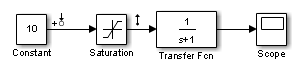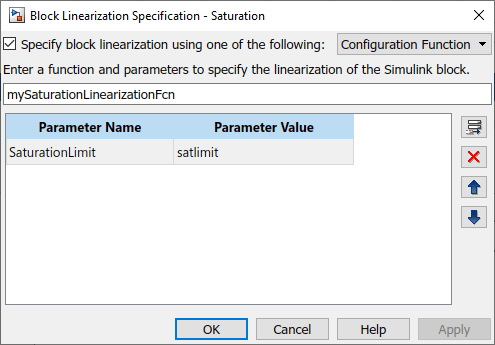# Specify D-Matrix System for Block Linearization Using Function

This example shows how to specify custom linearization for a saturation block using a function.

```mdl = 'configSatBlockFcn'; open_system(mdl)```In this model, the limits of the saturation block are `–satlimit` and `satlimit`.

Define the saturation limit, which is a parameter required by the linearization function of the Saturation block.

`satlimit = 10;`

Linearize the model at the model operating point using the linear analysis points defined in the model. Doing so returns the linearization of the saturation block.

```io = getlinio(mdl); linsys = linearize(mdl,io)```
```linsys = D = Constant Saturation 0.5 Static gain. ```

At the model operating point, the input to the saturation block is 10. This value is on the saturation boundary. At this value, the saturation block linearizes to 1.

Suppose that you want the block to linearize to a transitional value of 0.5 when the input falls on the saturation boundary. Write a function that defines the saturation block linearization to behave this way. For this example, use the configuration function in `mySaturationLinearizationFcn.m`.

```function blocklin = mySaturationLinearizationFcn(BlockData) % This function customizes the linearization of a saturation block. The % linearization of the block is as follows % BLOCKLIN = 0 when |U| > saturation limit % BLOCKLIN = 1 when |U| < saturation limit % BLOCKLIN = 0.5 when U = saturation limit % Get the saturation limit specified in the parameters of the block. satlimit = BlockData.Parameters.Value; % Compute the linearization based on the input signal level to the block. if abs(BlockData.Inputs.Values) > satlimit blocklin = 0; elseif abs(BlockData.Inputs.Values) < satlimit blocklin = 1; else blocklin = 0.5; end ```

This configuration function defines the saturation block linearization based on the level of the block input signal. For input values outside the saturation limits, the block linearizes to zero. Inside the limits, the block linearizes to 1. For boundary values, the block linearizes to the interpolated value of 0.5. The input to the function, BlockData, is a structure that the software creates automatically when you configure the linearization of the Saturation block to use the function. The configuration function reads the saturation limits from that data structure.

The input to the function, `BlockData`, is a structure that the software creates automatically each time it linearizes the block. When you specify a block linearization configuration function, the software automatically passes `BlockData` to the function. If your configuration function requires additional parameters, you can configure the block to set those parameters in the `BlockData.Parameters` field.

Specify `mySaturationLinearizationFcn` as the linearization for the Controller block.

1. In the Simulink model, right-click the Saturation block, and select Linear Analysis > Specify Selected Block Linearization.

2. In the Block Linearization Specification dialog box, select Specify block linearization using one of the following.

3. In the drop-down list, select `Configuration Function`.

4. In the text box, enter the function name `mySaturationLinearizationFcn`.

5. Specify the saturation limit as a parameter for the function. In the Parameter Value column, enter the variable name `satlimit`.

6. In the Parameter Name column, enter the corresponding descriptive name `SaturationLimit`.

7. Click OK.Alternatively, you can define the configuration function programmatically.

```satblk = 'configSatBlockFcn/Saturation'; set_param(satblk,'SCDEnableBlockLinearizationSpecification','on') rep = struct('Specification','mySaturationLinearizationFcn',... 'Type','Function',... 'ParameterNames','SaturationLimit',... 'ParameterValues','satlimit'); set_param(satblk,'SCDBlockLinearizationSpecification',rep)```

Linearize the model again. Now, the linearization uses the custom linearization of the saturation block.

`linsys_cust = linearize(mdl,io)`
```linsys_cust = D = Constant Saturation 0.5 Static gain. ```

At the model operating point, the input to the saturation block is 10. Therefore, the block linearizes to 0.5, the linearization value specified in the configuration function.